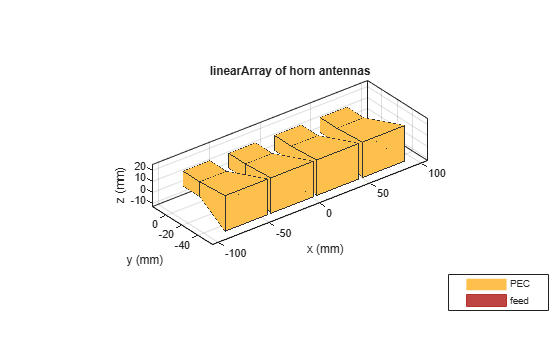Analyze Cylindrical Reflector Antenna with Horn Array Feed

This example shows how to analyze an antenna consisting of a cylindrical reflector with a linear array of horns.

Create Horn Antenna

Create a horn antenna resonating at 10 GHz. Set lambda to the free space wavelength at the design frequency of 10 GHz.

freq = 10e9;
lambda = physconst('light')/freq;
h = design(horn,10e9);
h.FlareWidth = 0.0416;
h.FlareLength = 0.0416;
h.FlareHeight = 31.8e-3;
h.Length = 21e-3;
h.FeedOffset(1) = -3.6e-3;
figure;
show(h)Plot VSWR and Radiation Pattern of Horn Antenna

Plot the voltage standing wave ratio (VSWR) of the horn antenna over the frequency range of 8.5–12.5 GHz.

range = (0.85:0.01:1.25)*freq;
figure;
vswr(h,range);
ylim([0 5]);The VSWR of the horn antenna is less than 2 between 8.6–12.2 GHz.

Plot the radiation pattern of the horn antenna.

figure;
pattern(h,freq);The directivity of the antenna is 11.4 dB.

Create Linear Array of Horns

Create a linear array of horns. Set the number of elements in the array to 4 and the spacing between the elements to 1.5 times of lambda.

arr = linearArray('Element',h,'NumElements',4);
arr.Element.Tilt = -90;
arr.Element.TiltAxis = 'Z';
arr.ElementSpacing = 1.5*lambda;
figure;
show(arr)Create Cylindrical Reflector with Horn Array

Create a cylindrical reflector with the linear array of horns as the exciter. Tilt the exciter at an angle of 90 degrees along the Z-axis and 70 degrees along the Y-axis.

ref = reflectorCylindrical;
ref.GroundPlaneLength = 10.2*lambda;
ref.GroundPlaneWidth = 10.3*lambda;
ref.Spacing = 8.5*lambda;
ref.Tilt = 90;
ref.Exciter = arr;
ref.Exciter.Tilt = [90 70];
ref.Exciter.TiltAxis = ['Z','Y'];
figure;
show(ref)Mesh and Plot Radiation Pattern of Cylindrical Reflector with Horn Array

Mesh the reflector manually with the maximum edge length of lambda/10.

figure;
mesh(ref,'MaxEdgeLength',lambda/10);Load the solved reflector file refObj.mat.

figure;
pattern(ref,freq);
view([80 10]);You can also plot the radiation pattern without using the MAT file, but the pattern(ref,freq) command takes up to 18 minutes to plot the radiation pattern. To run the pattern(ref,freq) command, these machine configurations are preferred:

• Processor: Intel ® Xeon ® CPU E5-1650 v4 @3.60GHz.

• RAM: 64GB.

• System type: 64-bit operating system.

The directivity of the cylindrical reflector antenna with a horn array feed is 18.7 dB.